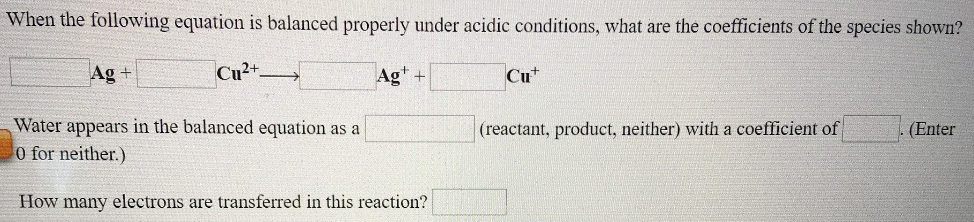# When the following equation is balanced properly under acidic conditions, what are the coefficients of the species shown? _____ Ag+ _____ Cu^2+ → _____Ag^+ + _____Cu^+ Water appears in the balanced equation as a ______ (reactant, product, neither) with a coefficient of _____. (Enter 0 for neither.) How many electrons are transferred in this reaction?